# Practice Applying Projectile Motion Formulas

Lesson Transcript
Instructor: Artem Cheprasov

Artem has a doctor of veterinary medicine degree.

In this lesson, you'll have a chance to practice real-world applications of projectile motion formulas. You'll learn how to approach these problems and we'll walk you through solving them.

## What Is Projectile Motion?

What do raindrops falling from the sky and a baseball flying through the air have in common? Projectile motion!

Projectile motion can be seen as motion in which an object is moving through space only under the influence of the force of gravity. This moving object can be of any size, shape, and material composition, and it is itself referred to as a projectile.

Although in many real-world situations other small forces may be involved, such as air resistance retarding a baseball's motion, we assume these other forces to be negligible for simplicity's sake.

Let's look at a few examples of applying projectile motion formulas to real-world problems.

The general strategy for solving projectile motion problems is to read through the problem making sure you understand what's happening, write down the known and unknown quantities, and solve for one unknown quantity at a time by selecting an equation that has the unknown quantities you're searching for, but not others.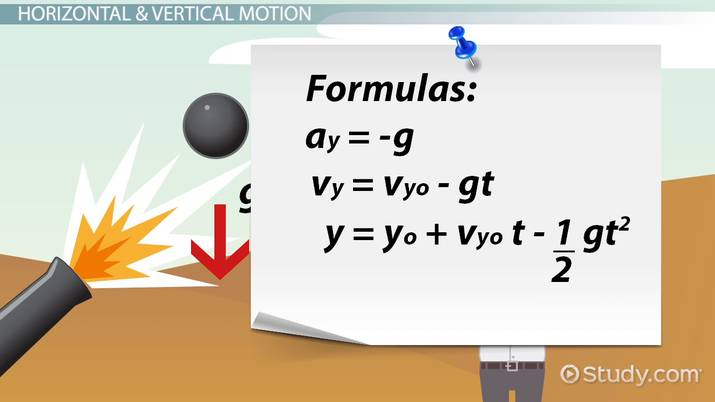An error occurred trying to load this video.

Try refreshing the page, or contact customer support.

Coming up next: Practice Applying Rotational Motion Formulas

### You're on a roll. Keep up the good work!

Replay
Your next lesson will play in 10 seconds
• 0:00 What Is Projectile Motion?
• 1:03 Horizontal & Vertical Motion
• 3:35 The Cannon Ball…
• 6:33 Eiffel Tower Practice Problem
• 8:52 Lesson Summary
Save Save

Want to watch this again later?

Timeline
Autoplay
Autoplay
Speed Speed

## Horizontal & Vertical Motion

To solve projectile motion problems, we have to keep a few things in mind. First, the projectile's horizontal velocity must remain constant, since gravity is only acting in the vertical direction. This leads us to the following formulas: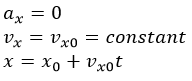The top equation tells us that the horizontal acceleration is zero. Consequently, the horizontal velocity at any time is constant and equal to the initial horizontal velocity, as shown in the second equation. In the bottom formula, x, the horizontal travel distance, is equal to the initial horizontal distance, xo, plus the product of the horizontal velocity, vo, and travel time, t.

How about in the vertical direction? Well, since we now have to account for the downward force of gravity, the formulas are as follows: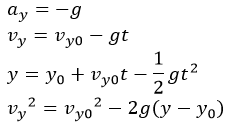The vertical acceleration has a constant value of minus g, where g is the acceleration due to gravity, 9.8 meters per second-squared on our planet. The second formula tells us that the final vertical velocity, vy, is equal to the initial vertical velocity, vo, minus g times t.

In the third equation, we have the final vertical position, y, equal to the initial vertical position, yo, plus vyo times t minus one-half times g times t squared. The final vertical velocity squared, vy squared, is equal to the initial vertical velocity squared, vo squared, minus 2 times g times the quantity y minus yo.

With that potentially boring, but still important, stuff out of the way, let's get to some cool examples. Just make sure to try each problem by yourself before seeing the solution this lesson provides. It's called practice for a reason!

## The Cannon Ball Practice Problem

Imagine firing a cannonball from a 60-meter-high hill onto the ground below. Assume that the cannonball's velocity is 25 m/s, that it is fired at a 40 degree angle with respect to the horizontal, and that you've ensured no one in the ground below.

Calculate the horizontal distance it will travel, its maximum height, and the total travel time until the cannonball hits the ground, as shown in the following illustration: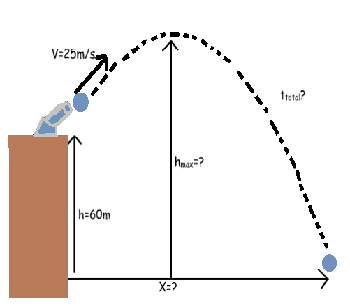For each of the unknowns we're solving for (time, max height, and the total horizontal range), the trick is to pick an equation that contains only one unknown. You can see that there are several different knowns in our example: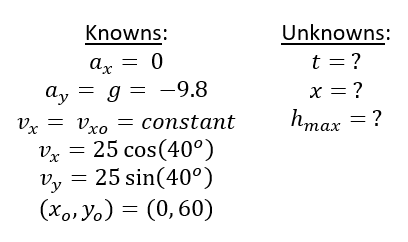The total travel time can be calculated using this equation because it contains the unknown t but not the unknowns x or hmax: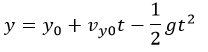Plugging in 60 for the initial vertical height, 25 times the sine of 40 degrees for the initial vertical velocity, and 9.8 for g, we obtain the quadratic equation: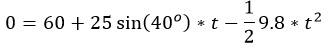After solving the quadratic equation, you should get t equals 5.5 seconds.

To unlock this lesson you must be a Study.com Member.

### Register to view this lesson

Are you a student or a teacher?